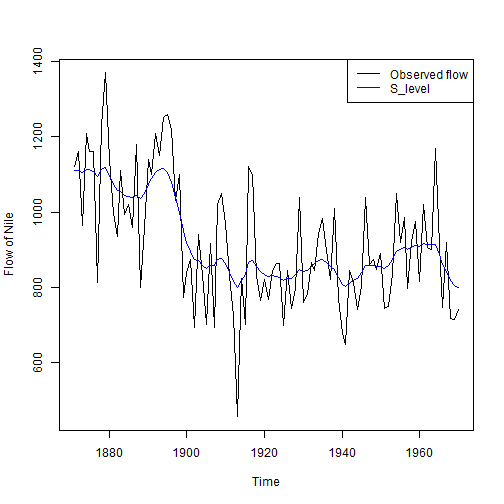Unobserved Component Models in R

Introduction

Unobserved Components Model (UCM) (Harvey (1989)) performs a time series decomposition into components such as trend, seasonal, cycle, and the regression effects due to predictor series. It can be represented as follows:

\begin{aligned} y_{t} & = \mu_{t} + \gamma_{t} + \psi_{t} + \sum_{j=1}^{m} \beta_{j} x_{jt} + \epsilon_{t} \end{aligned}

\begin{aligned} \epsilon_{t} & \sim i.i.d. N(0, \sigma^2_{\epsilon}) \end{aligned}

The components $$\mu_{t}$$, $$\gamma_{t}$$, and $$\psi_{t}$$ represent the trend, seasonal, and cyclical components, respectively; the term $$\sum_{j=1}^{m} \beta_{j} x_{jt}$$ gives the contribution of regression variables with fixed or time varying regression coefficients.

Trend

Trends are loosely defined as the natural tendency of the series to increase or decrease or remain constant over a period of time in absense of any other influencing variable.

UCM can model trend in two ways; first being the random walk model implying that trend remains roughly constant over the time period of the series, and the second being locaaly linear trend having an upward or downward slope.

Cycle

Cycles in a time series data exists when the data exhibit rises and falls that are not of fixed period. The duration of these fluctuations is usually of at least 2 years.

Seasonality

A seasonal pattern exists when there exists a consistent pattern of variation influenced by seasonal factors (e.g., the quarter of the year, or day of the week, etc.).

For a detailed discussion of all the above three factors see references below.

rucm-Package

Package rucm has been authored keeping in mind the easier specification of UCM in SAS using PROC UCM. rucm provides a wrapper function called ucm containing arguments specifying the formula for predictor variables, and other decomposition components such as level, slope, season, cycle, etc. to run UCM. ucm can also handle cases where we want to fix the variance of any of the above decomposition components.

To install rucm:

#install.packages("rucm")
library(rucm)

For help and the list of values that are returned see the help(package = rucm) or ?ucm.

Here we work with the Nile data which comes along with the datasets package, measures the annual flow of the river Nile at Ashwan, in south Egypt, between 1871 and 1970.

To model the level of Nile annual flow:

modelNile <- ucm(formula = Nile~0, data = Nile, level = TRUE)
modelNile #Printing method for class ucm
## Call:
## ucm(formula = Nile ~ 0, data = Nile, level = TRUE)
##
## Parameter estimates:
## NULL
##
## Estimated variance:
## Irregular_Variance     Level_Variance
##          15098.517           1469.175
plot(Nile, ylab = "Flow of Nile")
lines(modelNile$s.level, col = "blue") legend("topright", legend = c("Observed flow","S_level"), col = c("black","blue"), lty = 1)The formula argument in the ucm function takes an argument of the form as.formula in R. For multivariate UC Models, the rhs of the formula should contain the indepedent variables. If the model is univariate, we write a 0 in the rhs of the formula specification. Slope, seasonality, and cyclicity can be included by using slope = TRUE, season = TRUE, cycle = TRUE, specifying the seasonal and cyclical lengths of the series in the arguments season.length and cycle.period, respectively. ucm returns an object of class ucm having the estimate of predictors, estimated variances, time series of unobserved components (level, slope, whatever is included), and time series of the variances of these components. To forecast the time series, we use the predict function supplying the model name and number of periods to forecast in n.ahead. modelNile <- ucm(formula = Nile~0, data = Nile, level = TRUE, slope = TRUE) predict(modelNile$model, n.ahead = 12) # Forecasting
## Time Series:
## Start = 1971
## End = 1982
## Frequency = 1
##   779.5699 776.1597 772.7496 769.3395 765.9293 762.5192 759.1091
##   755.6989 752.2888 748.8786 745.4685 742.0584

References

1. Harvey A. (1989). Forecasting, structural time series models and the Kalman filter. Cambridge New York: Cambridge University Press

2. Helske J (2014). KFAS: Kalman filter and Smoothers for Exponential Family State Space Models. R package version 1.0.4-1, URL http://CRAN.R-project.org/package=KFAS.

3. Hyndsight. URL http://robjhyndman.com/hyndsight/cyclicts/.

4. SAS Institute Inc (2010). SAS/ETS 9.22 User's Guide. SAS Institute Inc., Cary, NC. URL http://support.sas.com/documentation/cdl/en/etsug/60372/PDF/default/etsug.pdf.

5. Selukar R (2011). “State Space Modeling Using SAS”. Journal of Statistical Software, 41(12), 1-13. URL http://www.jstatsoft.org/v41/i12/.

6. Petris G, Petrone S (2011). “State Space Models in R”. Journal of Statistical Software, 41(4), 1-25. URL http://www.jstatsoft.org/v41/i04/.